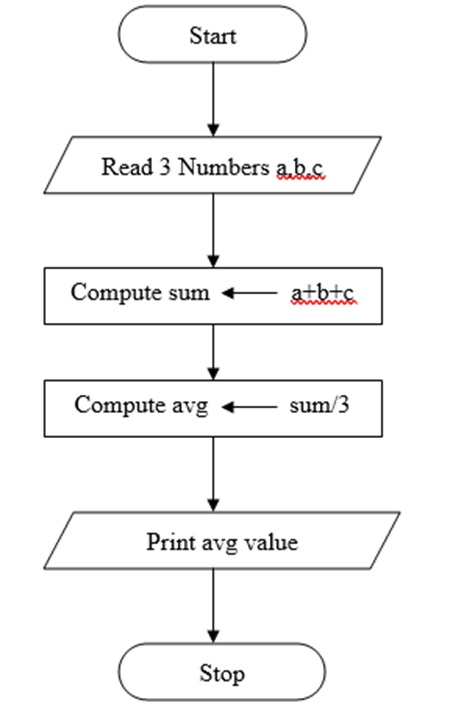# What is an algorithm and flowchart in C language?

CServer Side ProgrammingProgramming

Algorithm is a step – by – step procedure which is helpful in solving a problem. If, it is written in English like sentences then, it is called as ‘PSEUDO CODE’.

## Properties of an Algorithm

An algorithm must possess the following five properties −

• Input
• Output
• Finiteness
• Definiteness
• Effectiveness

## Example

Algorithm for finding the average of three numbers is as follows −

• Start
• Compute sum = a+b+c
• Compute average = sum/3
• Print average value
• Stop

## FLOW CHART

Diagrammatic representation of an algorithm is called flow chart.

Symbols used in flowchart are mentioned below −

NameSymbolPurpose
Terminal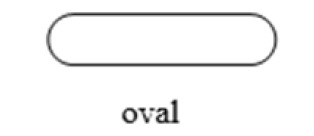Ovalstart/stop/begin/end
Input/output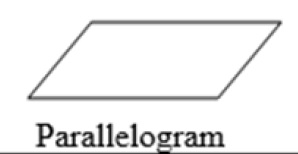Parallelogram
Input/output of data
Process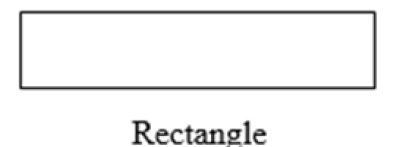RectangleAny processing to be performaed can be represented
Decision box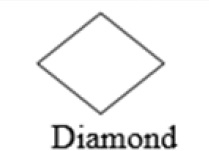DiamonDecision operation that determine which of the alternative paths to be followed
Connector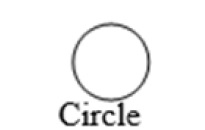CircleUsed to connect different parts of flowchart
Flow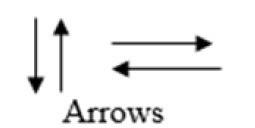ArrowsJoin 2 symbols and also represents flow of execution
Pre defined process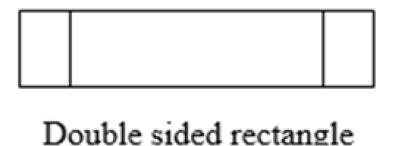Double Sided RectangleModule (or) subroutines specified else where
Page connector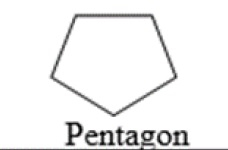PentagonUsed to connect flowchart in 2 different pages
For loop symbol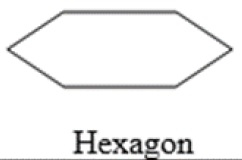Hexagonshows initialization, condition and incrementation of loop variable
Document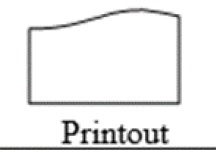PrintoutShows the data that is ready for printout

## Example

Given below is the flowchart for finding an average of three numbers −